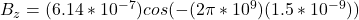A plane monochromatic radio wave (? = 0.3 m) travels in vacuum along the positive x-axis, with a time-averaged intensity I = 45.0 W/m2. Supp

Question

A plane monochromatic radio wave (? = 0.3 m) travels in vacuum along the positive x-axis, with a time-averaged intensity I = 45.0 W/m2. Suppose at time t = 0, the electric field at the origin is measured to be directed along the positive y-axis with a magnitude equal to its maximum value. What is Bz, the magnetic field at the origin, at time t = 1.5 ns? Bz = I got .04800 but that answer didnt work.

in progress 0
7 months 2021-07-15T19:57:58+00:00 1 Answers 50 views 0

The magnetic field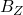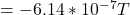Explanation:

From the question we are told that

The wavelength is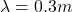The intensity is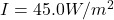The time is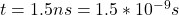Generally radiation intensity is mathematically represented as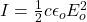Where  c is the speed of light with a constant value of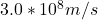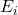is the electric field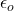is the permittivity  of free space with a constant value of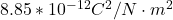Making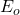the subject of the formula we have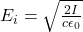Substituting values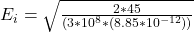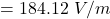Generally electric and magnetic field are related by the mathematical equation as follows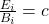Where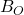is the magnetic field

makingthe subject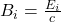Substituting values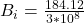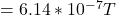Next is to obtain the wave number

Generally  the wave number is mathematically represented as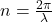Substituting values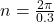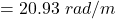Next is to obtain the frequency

Generally  the  frequency f is mathematically represented as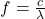Substituting values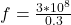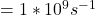Next is to obtain the angular velocity

Generally  the  angular velocity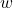is mathematically represented as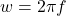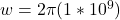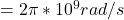Generally  the sinusoidal electromagnetic waves for the magnetic field B moving in the positive z direction is expressed as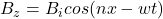Since the magnetic field is induced at the origin then the equation above is reduced to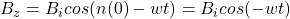x =0 because it is the origin we are considering

Substituting values